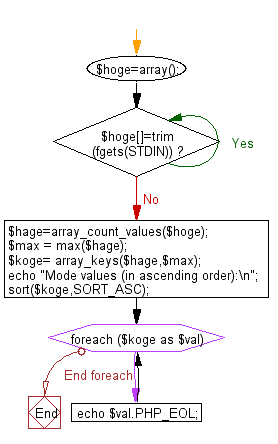﻿ PHP Exercise: Print mode values from a given a sequence of integers - w3resource

# PHP Exercises: Print mode values from a given a sequence of integers

## PHP: Exercise-60 with Solution

Write a PHP program to print mode values from a given a sequence of integers. The mode value is the element which occurs most frequently. If there are several mode values, print them in ascending order.

Input:A sequence of integer’s ai (1 ≤ ai ≤ 100). The number of integers is less than or equals to 100.

Sample Solution: -

PHP Code:

``````<?php
\$hoge=array();
while(\$hoge[]=trim(fgets(STDIN))){}
\$hage=array_count_values(\$hoge);
\$max = max(\$hage);
\$koge= array_keys(\$hage,\$max);
echo "Mode values (in ascending order):\n";
sort(\$koge,SORT_ASC);
foreach (\$koge as \$val) {
echo \$val.PHP_EOL;
}
?>
```
```

Sample Input:
6
7
4
6
9
8
6
4
10
8
4
5

Sample Output:

```Mode values (in ascending order):
4
6```

Flowchart:PHP Code Editor:

Have another way to solve this solution? Contribute your code (and comments) through Disqus.

What is the difficulty level of this exercise?

Test your Programming skills with w3resource's quiz.

﻿

## PHP: Tips of the Day

Returns all elements in an array except for the first one

Example:

```<?php
function tips_tail(\$items)
{
return count(\$items) > 1 ? array_slice(\$items, 1) : \$items;
}

print_r(tips_tail([1, 5, 7]));
?>
```

Output:

```Array
(
 => 5
 => 7
)
```